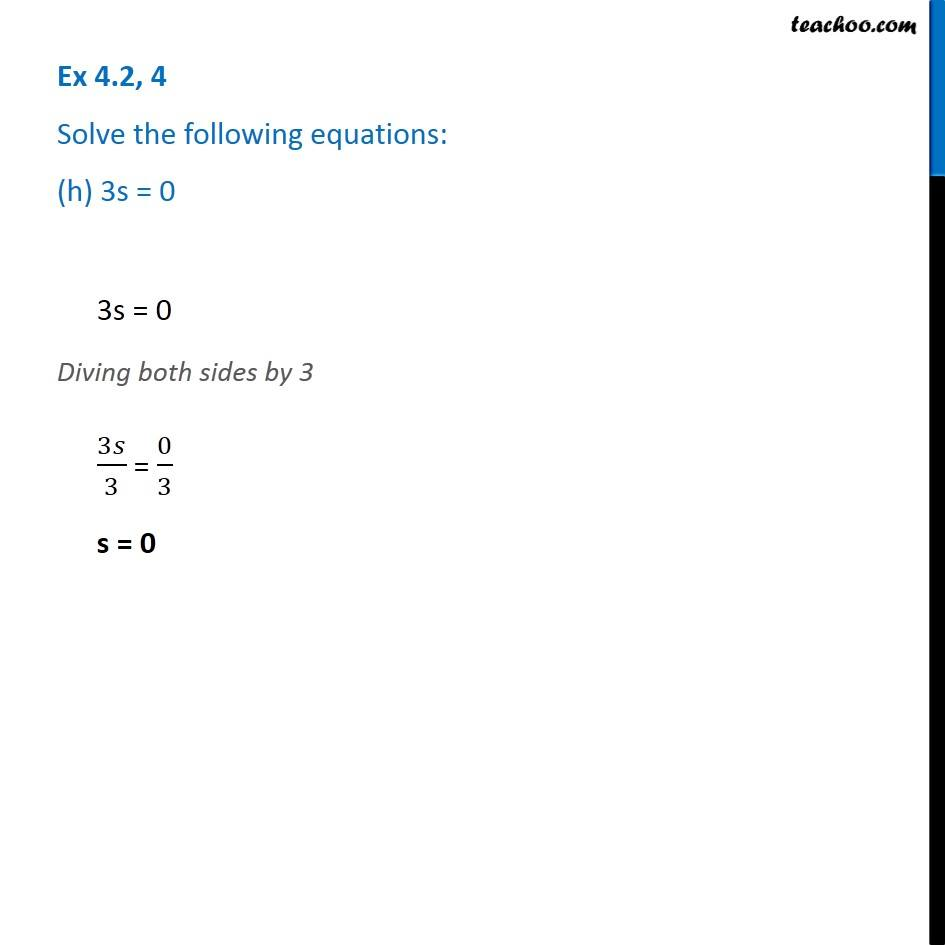1. Chapter 4 Class 7 Simple Equations
2. Serial order wise
3. Ex 4.2

Transcript

Ex 4.2, 4 Solve the following equations: (h) 3s = 03s = 0 Diving both sides by 3 3𝑠/3 = 0/3 s = 0